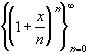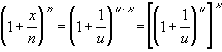#Interactive Real Analysis

Next | Previous | Glossary | Map

## 3.5. Special Sequences

### Definition 3.5.7: Exponential SequenceExponential Sequence: Converges to the exponential function ex = exp(x) for any real number x.

### Proof:

We will use a simple substitution to prove this. Let
x/n = 1/u, or equivalently, n = u x
Then we haveBut the term inside the square brackets is Euler's sequence, which converges to Euler's number e. Hence, the whole expression converges to ex, as required.

In fact, we have used a property relating to functions to make this proof work correctly. What is that property ?

If we did not want to use functions, we could first prove the statement for x being an integer. Then we could expand it to rational numbers, and then, approximating x by rational number, we could prove the final result.Next | Previous | Glossary | Map